Home
Hostname: page-component-cf9d5c678-xvx2z Total loading time: 0.205 Render date: 2021-07-27T13:22:14.387Z Has data issue: true Feature Flags: { "shouldUseShareProductTool": true, "shouldUseHypothesis": true, "isUnsiloEnabled": true, "metricsAbstractViews": false, "figures": true, "newCiteModal": false, "newCitedByModal": true, "newEcommerce": true, "newUsageEvents": true }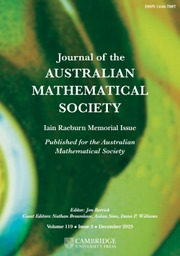Journal of the Australian Mathematical Society

# APPROXIMATELY MULTIPLICATIVE MAPS FROM WEIGHTED SEMILATTICE ALGEBRAS

Published online by Cambridge University Press:  17 June 2013

## Abstract

We investigate which weighted convolution algebras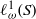${ \ell }_{\omega }^{1} (S)$, where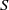$S$ is a semilattice, are AMNM in the sense of Johnson [‘Approximately multiplicative functionals’, J. Lond. Math. Soc. (2) 34(3) (1986), 489–510]. We give an explicit example where this is not the case. We show that the unweighted examples are all AMNM, as are all${ \ell }_{\omega }^{1} (S)$ where$S$ has either finite width or finite height. Some of these finite-width examples are isomorphic to function algebras studied by Feinstein [‘Strong Ditkin algebras without bounded relative units’, Int. J. Math. Math. Sci. 22(2) (1999), 437–443]. We also investigate when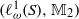$({ \ell }_{\omega }^{1} (S), { \mathbb{M} }_{2} )$ is an AMNM pair in the sense of Johnson [‘Approximately multiplicative maps between Banach algebras’, J. Lond. Math. Soc. (2) 37(2) (1988), 294–316], where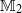${ \mathbb{M} }_{2}$ denotes the algebra of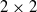$2\times 2$ complex matrices. In particular, we obtain the following two contrasting results: (i) for many nontrivial weights on the totally ordered semilattice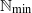${ \mathbb{N} }_{\min }$, the pair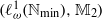$({ \ell }_{\omega }^{1} ({ \mathbb{N} }_{\min } ), { \mathbb{M} }_{2} )$ is not AMNM; (ii) for any semilattice$S$, the pair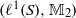$({\ell }^{1} (S), { \mathbb{M} }_{2} )$ is AMNM. The latter result requires a detailed analysis of approximately commuting, approximately idempotent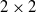$2\times 2$ matrices.

Type
Research Article
Information

## References

Birkhoff, G., Lattice Theory, 3rd edn, American Mathematical Society Colloquium Publications, 25 (American Mathematical Society, Providence, RI, 1979).Google Scholar
Dales, H. G. and Loy, R. J., ‘Approximate amenability of semigroup algebras and Segal algebras’, Dissertationes Math. (Rozprawy Mat.) 474 (2010), 58.Google Scholar
Feinstein, J. F., ‘Strong Ditkin algebras without bounded relative units’, Int. J. Math. Math. Sci. 22 (2) (1999), 437443.CrossRefGoogle Scholar
Ghahramani, F., Loy, R. J. and Zhang, Y., ‘Generalized notions of amenability, II’, J. Funct. Anal. 254 (7) (2008), 17761810.CrossRefGoogle Scholar
Hewitt, E. and Zuckerman, H. S., ‘The${l}_{1}$-algebra of a commutative semigroup’, Trans. Amer. Math. Soc. 83 (1956), 7097.Google Scholar
Howey, R. A. J., ‘Approximately multiplicative maps between some Banach algebras’, PhD thesis, The University of Newcastle-upon-Tyne, 2000.Google Scholar
Howey, R. A. J., ‘Approximately multiplicative functionals on algebras of smooth functions’, J. Lond. Math. Soc. (2) 68 (3) (2003), 739752.CrossRefGoogle Scholar
Jarosz, K., Perturbations of Banach Algebras, Lecture Notes in Mathematics, 1120 (Springer, Berlin, 1985).CrossRefGoogle Scholar
Jarosz, K., ‘Almost multiplicative functionals’, Studia Math. 124 (1) (1997), 3758.CrossRefGoogle Scholar
Johnson, B. E., ‘Approximately multiplicative functionals’, J. Lond. Math. Soc. (2) 34 (3) (1986), 489510.CrossRefGoogle Scholar
Johnson, B. E., ‘Approximately multiplicative maps between Banach algebras’, J. Lond. Math. Soc. (2) 37 (2) (1988), 294316.CrossRefGoogle Scholar
Lawrence, J., ‘The stability of multiplicative semigroup homomorphisms to real normed algebras. I’, Aequationes Math. 28 (1–2) (1985), 94101.CrossRefGoogle Scholar
N.N., ‘personal communication’, http://mathoverflow.net/questions/100971 (version: 2012-06-29), MathOverflow, 2012.Google Scholar
Sidney, S. J., ‘Are all uniform algebras AMNM?’, Bull. Lond. Math. Soc. 29 (3) (1997), 327330.CrossRefGoogle ScholarYou have Access
2
Cited by

# Send article to Kindle

Note you can select to send to either the @free.kindle.com or @kindle.com variations. ‘@free.kindle.com’ emails are free but can only be sent to your device when it is connected to wi-fi. ‘@kindle.com’ emails can be delivered even when you are not connected to wi-fi, but note that service fees apply.

Find out more about the Kindle Personal Document Service.

APPROXIMATELY MULTIPLICATIVE MAPS FROM WEIGHTED SEMILATTICE ALGEBRAS
Available formats
×

# Send article to Dropbox

To send this article to your Dropbox account, please select one or more formats and confirm that you agree to abide by our usage policies. If this is the first time you use this feature, you will be asked to authorise Cambridge Core to connect with your <service> account. Find out more about sending content to Dropbox.

APPROXIMATELY MULTIPLICATIVE MAPS FROM WEIGHTED SEMILATTICE ALGEBRAS
Available formats
×

# Send article to Google Drive

To send this article to your Google Drive account, please select one or more formats and confirm that you agree to abide by our usage policies. If this is the first time you use this feature, you will be asked to authorise Cambridge Core to connect with your <service> account. Find out more about sending content to Google Drive.

APPROXIMATELY MULTIPLICATIVE MAPS FROM WEIGHTED SEMILATTICE ALGEBRAS
Available formats
×
×